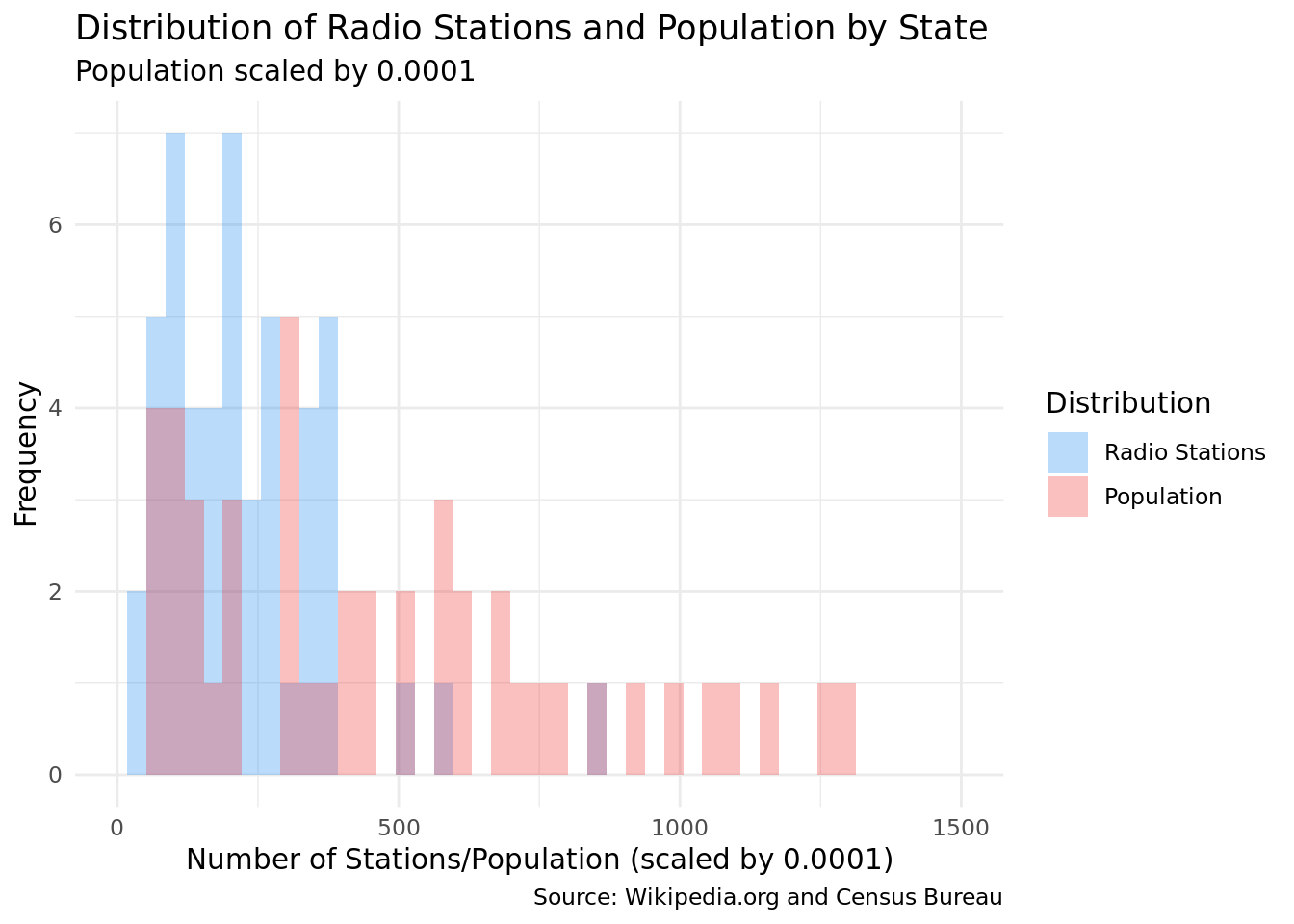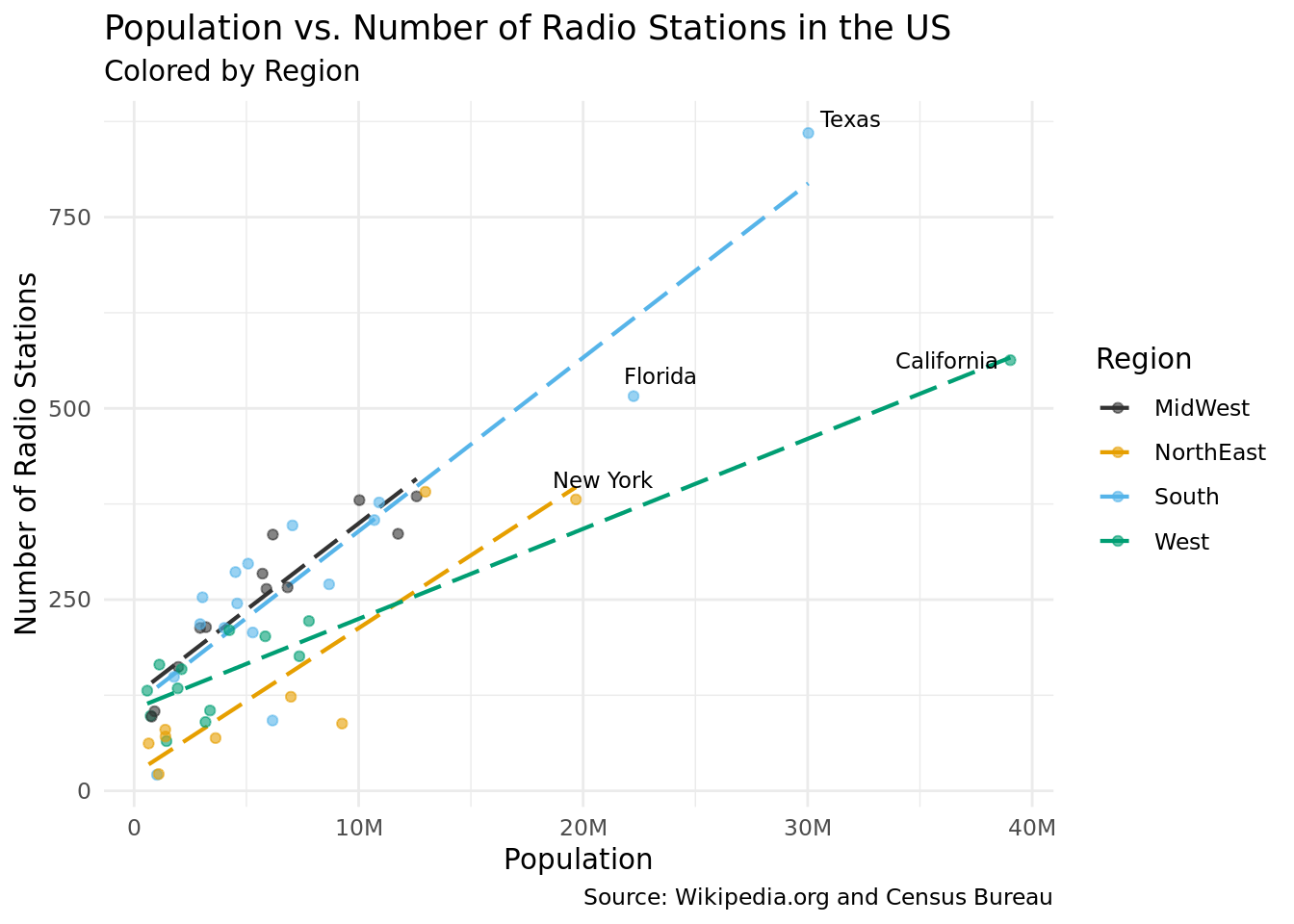# Exploring the Distribution of Radio Stations Across the U.S.

STA/ISS 313 - Project 1

Author

Stats FM

## Abstract

This project aims to examine trends in radio stations across the United States. It explores if there are regional trends in the genre of radio stations in each state and also studies the relationship between population density and the number of radio stations in each state. We used various plotting methods to analyze potential trends in our data. We concluded that there was not a strong trend in the data regarding the relationship between region and genre. In addition, there is potential that a higher population is correlated to the number of radio stations in a state, but our results were not extremely conclusive.

## Introduction

This dataset contains information concerning radio stations in all 50 states of the United States. The data was mined from the “Lists of Radio Stations in the United States” Wikipedia page in 2022 and contains information such as the abbreviated name or call sign of the radio station (`call_sign`), the channel (`frequency`), the licensee (`licensee`), the city where the station is located (`city`), the kind of content of the radio station (`format`), and the state where the radio station is located (`city`). All of these variables are categorical variables, and we will use external data to further analyze trends in our data.

## Question 2: Identifying correlation between population size and the number of radio stations in various states/regions of the United States

### Introduction

Population size and density varies across the United States. For example, according to World Population Review (https://worldpopulationreview.com/state-rankings/state-densities), in 2023 Wyoming had a population of 581,075 and a population density of about 6 people per square mile. Meanwhile, New York has a population of 19,300,000 people and a population density of about 410 people per square mile. Given this radical variability in population and population density, it may be possible to find a correlation between these quantities and the variability in the number of radio stations in each state. Exploring this relationship may help us understand how a population or population density influences the number of radio stations that exist in a particular state or region. We will be using data from the state-stations dataset and the census population dataset. We will need the `state`, `population` and `region` variables along with a variable to represent the number of radio stations in a state for these visualizations.

### Approach

In the first plot we are going to investigate the relationship between the population and the number of radio stations by state. To address this question, we are going to layer the two distributions using a histogram plot where x-axis is the number of radio stations and the population size scaled by 0.0001. Histograms can help us easily see the frequency and overall distribution of each to identify interesting characteristics in the shape, spread, outliers, etc.

To further explore this relationship, we will need to create a scatterplot that plots each state as a point where the x-axis represents the population of a state and the y-axis represents the number of radio stations in the state. Each point will be color-coded to a particular region of the US based on the state. The scatter plot will be an effective visualization because it makes it easy to identify trends based on the distribution of all 50 data points. Identifying the region of points by color will help us search for unique trends in the relationship between population and radio stations based on region. We will be using a dataset where each row represents a radio station and we will only need the ‘state’ variable. We will also be pulling data from the dataset on population in the US by state, where we will need to utilize the ‘population’ state and region variables. Our approach will require use to mutate the data on the radio station dataset so that it only provides the ‘count’ (number of radio stations) and the state corresponding to that value. We will then join this dataset with the population dataset. We will also mutate the ‘region’ variable, where we will assign a region to a row based on the ‘state’. From here, we will use ggplot to render our visualization with a scatterplot, a linear regression for each region and text labels of outlier data points.

### Analysis### Discussion

It is hard to identify a relation between the two variables because the distribution of the number of radio stations seems to be different to the distribution of population size in US states. Both distributions show a right skew (population more so than radio stations) and the population variable appears to have a wider spread. There is a concentration of states with a population size around 250 on the x-axis (scaled by 0.0001, so approximately 2,500,000). Similarly, there is also a concentration of states with around 250 radio stations, meaning that for the left area of the graph, the number of radio stations by state could possibly have a relationship with the population size. However, we see that as the population size increases, the number of radio stations per state does not necessarily increase in relation. The population histogram extends to around 1,300 (which when scaled back is 13,000,000). We removed outliers (such as Texas and California) that would have extended the distribution further. These are further explored in the second plot.

The scatter plot reveals a few interesting trends in the data. The first and most obvious trend we can spot is that there is a positive correlation between a state’s population and the number of radio stations in a state. This trend is consistent across all regions of the US. We speculate that his trend exists because states with higher populations may tend to have a higher demand for radio stations. Another interesting trend that we identified was that southern and midwestern states tend to have a higher number of radio stations than north-eastern states given a particular population. One potential explanation for this is that southern and midwestern states have a higher demand for specific types of radio stations. For example, Alabama, a very religious state, may have a higher demand for religious radio stations than New Hampshire, a less religious state. Another possibility is that southern and midwestern states have larger rural populations, which may require the use of more radio stations to reach the isolated populus.